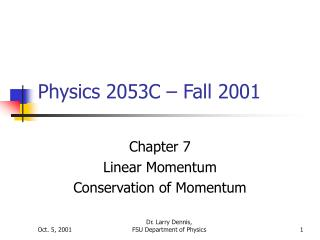DownloadDownload PresentationPhysics 2053C – Fall 2001

# Physics 2053C – Fall 2001

Télécharger la présentation## Physics 2053C – Fall 2001

- - - - - - - - - - - - - - - - - - - - - - - - - - - E N D - - - - - - - - - - - - - - - - - - - - - - - - - - -
##### Presentation Transcript

1. Physics 2053C – Fall 2001 Chapter 7 Linear Momentum Conservation of Momentum Dr. Larry Dennis, FSU Department of Physics

2. Conservation of Momentum • Recall: • Generalized Work-Energy Theorem K1 + U1 + Wnc = K2 + U2 Conservation of Energy means the total energy doesn’t change. • Conservation of Momentum means the total momentum doesn’t change. What’s Momentum????

3. Momentum p = mv • Momentum = mass * velocity • Momentum is a vector. • It is parallel to the velocity.

4. Equivalent Formulation of Newton’s Second Law The rate of change of momentum of a body is equal to the net force applied to it.

5. Equivalent Formulation of Newton’s Second Law The rate of change of momentum of a body is equal to the net force applied to it.

6. Newton’s Second Law & Momentum • When there is a net force: Momentum changes. • p =  Ft = Impulse • When there is no net force: Momentum remains constant. • p =  Ft = 0

7. CAPA 1 & 2 • A golf ball of mass 0.05 kg is hit off the tee at a speed of 45 m/s. The golf club was in contact with the ball for 5.0x10-3 s. • Find the impulse imparted to the ball. Impulse = Ft = p = mvf – mvo Impulse = m(vf – vo) = 0.05kg * (45 – 0)m/s Impulse = 2.25 kg-m/s = 2.25 N-s

8. CAPA 1 & 2 • A golf ball of mass 0.05 kg is hit off the tee at a speed of 45 m/s. The golf club was in contact with the ball for 5.0x10-3 s. • Find the average force imparted to the golf ball by the club. Impulse = Ft ( = 2.25 N-s ) Impulse/t = F F = 2.25 N-s/5.0x10-3 s = 450 N

9. Application to Collisions When p= 0 then: Momentum Before Collision = Momentum After Collision Mathematically this means: M1V1b + M2V2b = M1V1a + M2V2a

10. Types of Collisions An Elastic Collision – Kinetic Energy does not change. An Inelastic Collision – Kinetic Energy changes

11. Inelastic Collision: CAPA 4 Momentum Before Collision = Momentum After Collision MtVtb + MsVsb = MtVta + MsVsa Vtb Vsb Vta = Vsa = 0 MtVtb + MsVsb = (Mt+Ms) 0 MtVtb = - MsVsb Vsb = -Vtb*Mt/Ms

12. Inelastic Collision Momentum Before Collision = Momentum After Collision M1V1b + M2V2b = M1V1a + M2V2a V1b = Vb V2b = 0 V1a = V2a = Va M1Vb + M20 = (M1+M2)Va

13. Vb Va Inelastic Collisions (cont) M1Vb + M20 = (M1+M2)Va M1Vb = (M1+M2)Va M1/(M1+M2) Vb = Va

14. V1b V2b U1b U2b Center of Mass Velocity M1V1b + M2V2b (M1 + M2 )Vcm U = V - Vcm and V = U + Vcm

15. U1b U2b Elastic Collision in the CM U2a U1a M1U1a + M2U2a = 0 M1U1b + M2U2b = 0 ½M1U21b + ½M2U22b = ½M1U21a + ½M2U22a

16. Elastic Collision in the CM M1U1b + M2U2b = 0 M1U1a + M2U2a = 0 ½M1U21b + ½M2U22b = ½M1U21a + ½M2U22a Solution – Mirror Image: U1a= -U1b U2a= -U2b

17. 3.88 m/s 4.23 m/s Elastic Collision in the CM: CAPA #6-9 • A pair of bumper cars in an amusement park ride collide elastically as one approaches the other directly from the rear. One has mass m1 = 467 kg and the other mass m2 = 567 kg. If the lighter one approaches at v1 = 4.23 m/s and the other one is moving at v2 = 3.88 m/s, calculate: • the velocity of the lighter car after the collision. • the velocity of the heavier car after the collision. • the change in momentum of the lighter car. • the change in momentum of the heavier car.

18. 3.88 m/s Elastic Collision in the CM: CAPA #6-9 • Calcuate Vcm = (M1V1b+M2V2b)/(M1+M2) • Calcuate U1b and U2b • U1b = V1b – Vcm and U2b = V2b – Vcm • Set U1a = -U1b and U2a = -U2b • Calcuate V1a and V2a • V1a = U1a + Vcm and V2a = U2a + Vcm

19. U2b U1b U1a U2a Elastic Collision in the CM: CAPA #6-9 • Calcuate Vcm = (M1V1b+M2V2b)/(M1+M2) • Calcuate U1b and U2b • U1b = V1b – Vcm and U2b = V2b – Vcm Vcm = (M1V1b+M2V2b)/(M1+M2) Vcm = (467*4.23 + 567*3.88)/(467+567) = 4.038 m/s U1b = V1a - Vcm = 4.23 m/s - 4.038 m/s = 0.192 m/s U2b = V2b - Vcm = 3.88 m/s - 4.038 m/s = -0.158 m/s

20. U2b U1b U1a U2a Elastic Collision in the CM: CAPA #6-9 • Set U1a = -U1b and U2a = -U2b • Calcuate V1a and V2a • V1a = U1a + Vcm and V2a = U2a + Vcm U1a = - U1b = -0.192 m/s U2a = -U2b = 0.158 m/s V1a = U1a + Vcm = -0.192 m/s + 4.038 m/s = 3.846 m/s V2a = U2a + Vcm = 0.158 m/s + 4.038 m/s = 4.196 m/s 4.20 m/s 3.85 m/s

21. Elastic Collision in the CM: CAPA #6-9 Impulse = mVa – mVb (for each of cars 1 and 2) Note: Impulse on car 1 = - Impulse on car 2.

22. Next Time • Chapter 7 – Conservation of Momentum. • Quiz on Chapter 7. • Please see me with any questions or comments. See you on Monday.